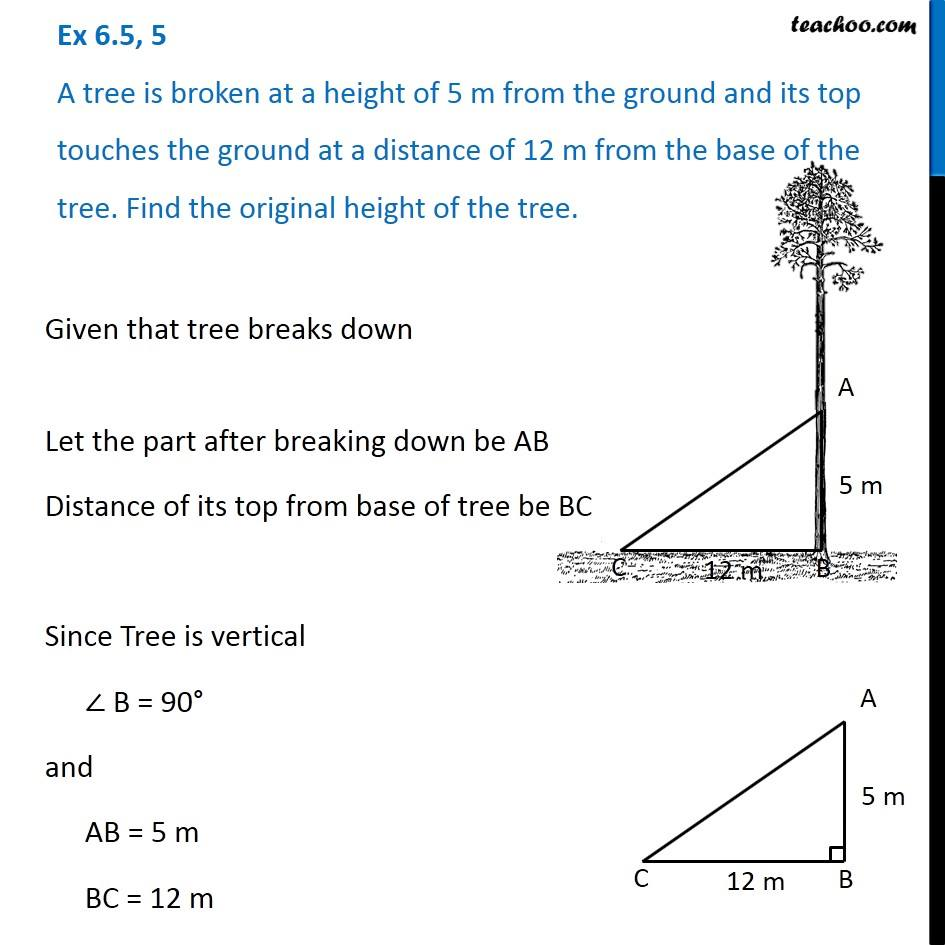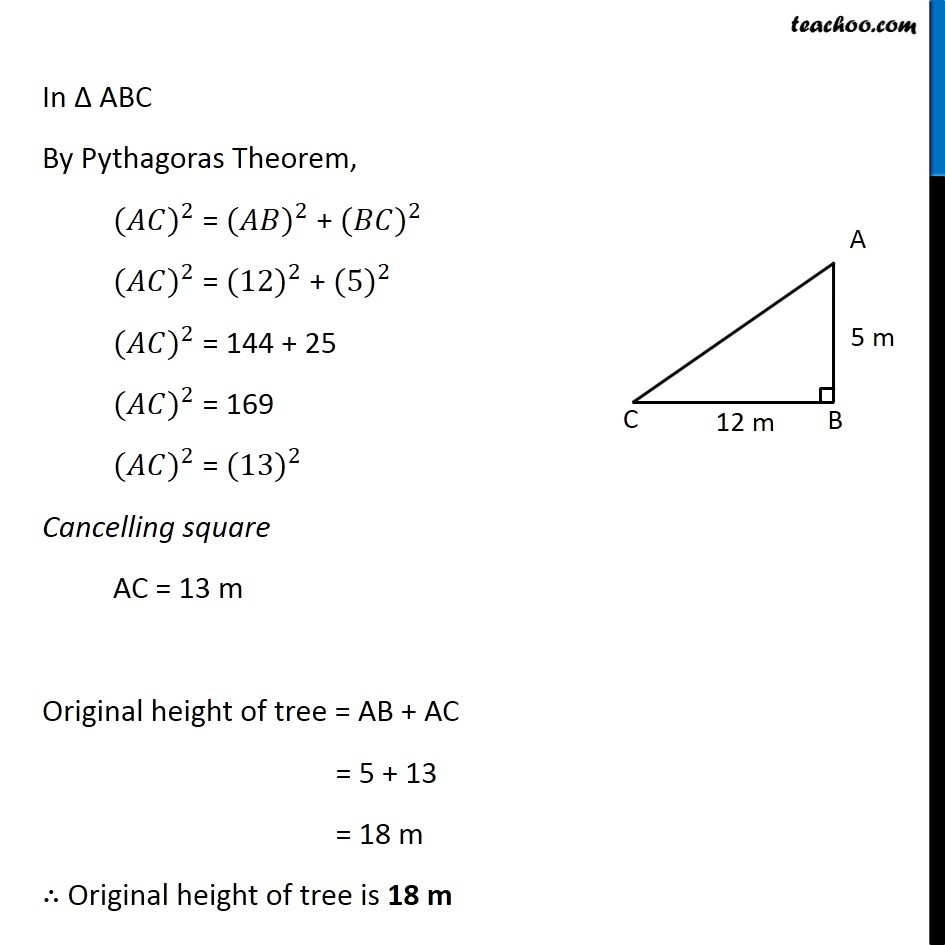Ex 6.5

Chapter 6 Class 7 Triangle and its Properties
Serial order wiseLearn in your speed, with individual attention - Teachoo Maths 1-on-1 Class

### Transcript

Ex 6.5, 5 A tree is broken at a height of 5 m from the ground and its top touches the ground at a distance of 12 m from the base of the tree. Find the original height of the tree. Given that tree breaks down Let the part after breaking down be AB Distance of its top from base of tree be BC Since Tree is vertical ∠ B = 90° and AB = 5 m BC = 12 m In Δ ABC By Pythagoras Theorem, 〖(𝐴𝐶)〗^2 = 〖(𝐴𝐵)〗^2 + 〖(𝐵𝐶)〗^2 〖(𝐴𝐶)〗^2 = 〖(12)〗^2 + 〖(5)〗^2 〖(𝐴𝐶)〗^2 = 144 + 25 〖(𝐴𝐶)〗^2 = 169 〖(𝐴𝐶)〗^2 = 〖(13)〗^2 Cancelling square AC = 13 m Original height of tree = AB + AC = 5 + 13 = 18 m ∴ Original height of tree is 18 m